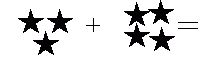Name: ___________________Date:___________________

 Email us to get an instant 20% discount on highly effective K-12 Math & English kwizNET Programs!

Grade 1 - Mathematics3.25 Computation Review Test

 Q 1: It does not matter what order you add numbers in, the sum is still the same.TrueFalse Q 2: Jan had 5 dolls. Her mother gave her 2 more. How many does she have in all?5786 Q 3: Find the missing operand.+- Q 4: Count the number of things and write the answer.Answer: Q 5: Double 6 you get1236 Q 6: Count the number of things and write the answer.Answer: Q 7: In the hundred table find the number 26 count 10. What is the answer?203036 Q 8: Write 25 + 26 in doubles plus one form.25 + 25 + 1 = 5125 + 26 + 1 = 5123 + 24 + 1 = 5124 + 25 + 2 = 51 Question 9: This question is available to subscribers only! Question 10: This question is available to subscribers only!